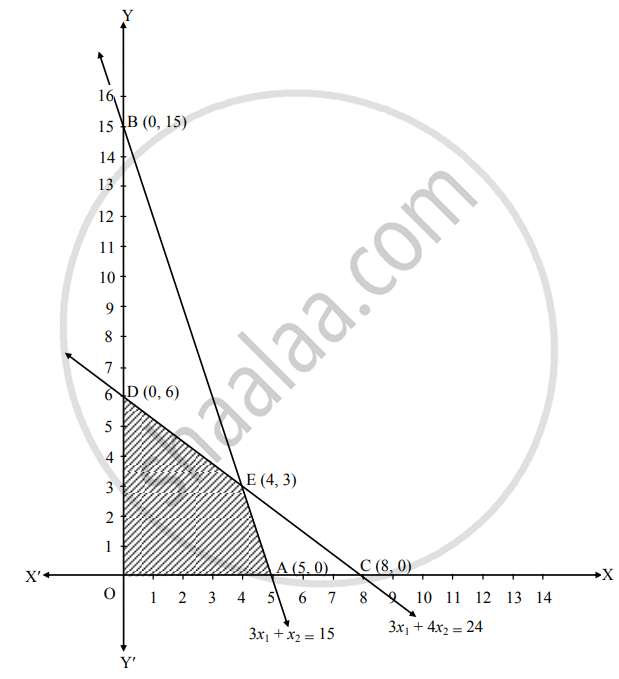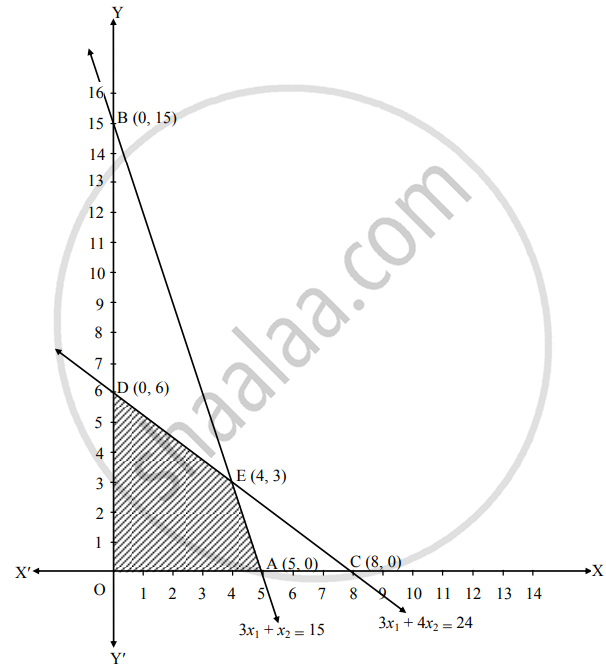# Solve the following problem : Maximize Z = 4x1 + 3x2 Subject to 3x1 + x2 ≤ 15, 3x1 + 4x2 ≤ 24, x1 ≥ 0, x2 ≥ 0 - Mathematics and Statistics

Graph
Sum

Solve the following problem :

Maximize Z = 4x1 + 3x2 Subject to 3x1 + x2 ≤ 15, 3x1 + 4x2 ≤ 24, x1 ≥ 0, x2 ≥ 0

#### Solution

To find the graphical solution, construct the table as follows:

 Inequation Equation Double intercept form Points (x1, x2) Region 3x1 + x2 ≤ 15 3x1 + x2 = 15 x_1/(5) + x_2/(15) = 1 A (5, 0)B (0, 15) 3(0) + 0 ≤ 15∴ 0 ≤ 15∴ origin side 3x1 + 4x2 ≤  24 3x1 + 4x2 = 24 x_1/8 + x_2/(6) = 1 C (8, 0)D (0, 6) 3(0 + 4(0) ≤ 24∴ 0 ≤ 24∴ origin side x1 ≥ 0 x1 = 0 – – R.H.S. of Y-axis x2 ≥ 0 x2 = 0 – – above X-axisShaded portion ODEA is the feasible region.
Whose vertices are O (0, 0), D (0, 6), E, A (5, 0)
E is the point of intersection of the lines
3x1 + x2 = 15               …(i)
and 3x1 + 4x2 = 24     …(ii)
∴ By (i) – (ii), we get
3x1 +   x2 = 15
3x1 + 4x2 = 24
–    –        –
–3x2 = – 9

∴ x2 = (-9)/(-3)

∴ x2 = 3
Substituting x2 = 3 in i, we get
3x1 + 3 = 15
∴ 3x1 = 15 – 3
∴ 3x1 = 12
∴ x1 = (12)/(3) = 4
∴ E (4, 3)
Here, the objective function is Z = 4x1 + 3x2
Now, we will find maximum value of Z as follows:

 Feasible points The value of Z = 4x1 + 3x2 O (0, 0) Z = 4(0) + 3(0) = 0 D (0, 6) Z = 4(0) + 3(0) = 0 E (4, 3) Z = 4(4) + 3(3) = 16 + 9 = 25 A (5, 0) Z = 4(4) + 3(3) = 16 + 9 = 25

∴ Z has maximum value 25 at E(4, 3)
∴ Z is maximum, when x1 = 4, x2 = 3.

Is there an error in this question or solution?
Chapter 6: Linear Programming - Miscellaneous Exercise 6 [Page 104]

#### APPEARS IN

Balbharati Mathematics and Statistics 2 (Commerce) 12th Standard HSC Maharashtra State Board
Chapter 6 Linear Programming
Miscellaneous Exercise 6 | Q 4.05 | Page 104
Share